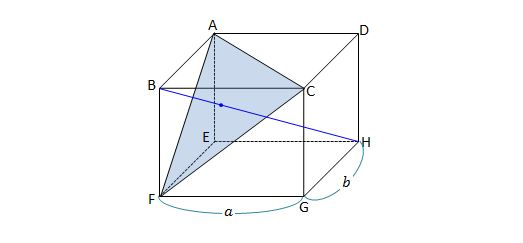Geometry

# 3D Section Formula

What are the coordinates of $P$ which divide the line segment joining the points $A=(-4,4,1)$ and $B=(4,-4,2)$ in the ratio $3:2 ?$

Let $A ( 4, 3, 5 )$ and $B ( 2, 6 , 0)$ be two fixed points. Find the position vector of the point which lies on the line segment $\overline{AB}$ and internally divides it in the ratio $7 : 8.$The above rectangular cuboid has base dimensions $a=12$ and $b=8.$ If the centroid $I$ of triangle $ACF$ internally divides the diagonal $\overline{BH}$ of the rectangular cuboid in the ratio $BI:IH=1:m,$ what is the value of $m?$

Suppose three vertices of a parallelogram are $O=(0,0,0), A=(1,5,6), B=(-1,-5,5),$ where $\overline{OA}$ and $\overline{OB}$ are adjacent sides. If $C$ is the final vertex of the parallelogram, then what are the coordinates of $C ?$

Consider a triangle $ABC$ such that the centroid of of the triangle is $G=(5,-2,3)$ and the midpoint of $\overline{AB}$ is $P=\left(1,\frac{1}{2},\frac{1}{2}\right).$ What are the coordinates of the point $C ?$

×